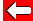# Simultaneous Equations

Suppose that we were given the following equations:

x + y = 25
3x = 15

We have two different equations with two unknowns - this is enough information for us to solve them both (that is, find the values of x and y). We call these simultaneous equations because we use them at the same time.

In the second equations, we can solve immediately for x (see the algebra pages if you're not happy with this):

3x = 15 so x = 5

Now we put this value of x (called substitution) into the first equation:

5 + y = 25 so y = 20

So the only correct solution for the two equations given at the top of the page is x = 5 and y = 20.Go back a pageMaths MenuGo to next page